# The model of artificial neuron and its general principles - Class review

Last updated on：4 months ago

There some basic principles of an artificial neuron. The content is mostly about the review of class “Optimal theories and methods”.

# Artificial neuron

An artificial neuron is a mathematical function conceived as a model of biological neurons. An artificial neuron is elementary units in an artificial neural network.
The artificial neuron receives one or more inputs and sums them to produce an output.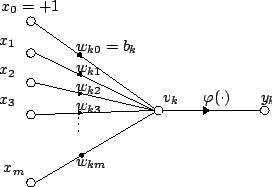# Components

Neural networks generally consist of five components:

• A directed graph is known as the network topography.
• A state variable associated with each neuron.
• A real-valued weight associated with each connection.
• A real-valued bias associated with each neuron.
• A transfer function $f$ for each neuron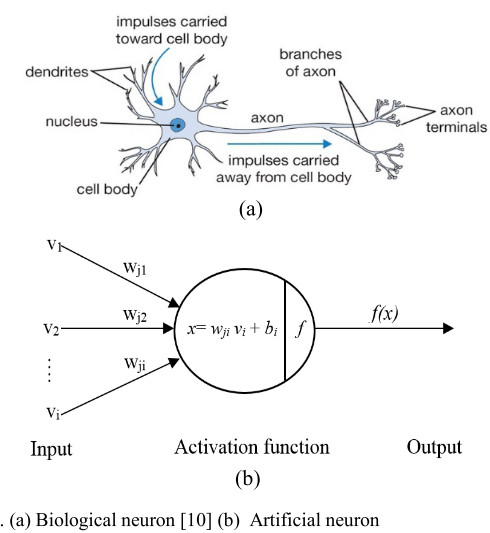$$\begin{cases} u_i = \sum^n_{j=1} w_{ij}s_j \ x_i = u_i - \theta_i \ v_i = f(x_i) \end{cases}$$

where $i = 1,2,…,n$.

## Input and output

On the last image, $x_1, x_2, …, and x_m$ is input (some features), and $y_i$ is output.

## Weights

When $w_{ij}>0$ means activation, by contrast, $w_{ij}<0$ means deactivation. The values of weights can measure the degree of impact between nodes $i$ and $j$ in a different layer.

## Activation function/Transfer function

The activation function of a node defines the output of that node given an input or set of inputs. Only nonlinear activation functions allow such networks to compute nontrivial problems using only a small number of nodes, and such activation functions are called nonlinearities.

Utilizing nonlinear activation functions can avoid linear units can be simplified as one unit. Nonlinear activation function can also be used to solve non-linearly separable problems.

$\phi$ is the transfer function (commonly a threshold function).

### Logistic function

The logistic function is a classic activation function always used in ANN. There are two types of logistic function which are sigmoid function and hyperbolic tangent function (tanh).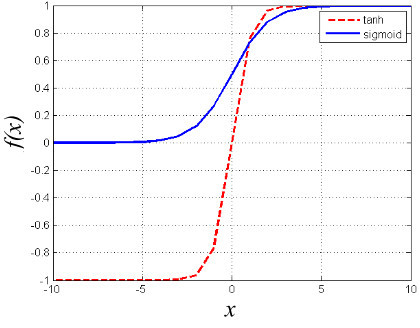#### Sigmoid function

The function is expressed as:

$$f_1 (x) = \frac{1}{1+exp(-ax)}$$

where $x=w_{ij}v_i+b_i$ and the slope parameter $a$.

Drawbacks:

• Vanishing gradient problem. The gradient for the data fallen in the region of either 0 or 1 is almost zero. Data is not passed the neurons to the update the weights parameter during BP training.
• The sigmoid activation function centre on 0.5. Thus, it slows down the learning process of the DNN.

#### tanh function

It is a rescaled and biased version of sigmoid function.

$$f_2 (x) = \frac{exp(2x) - 1}{exp(2x) + 1}$$

### Multistate activation function (MSAF)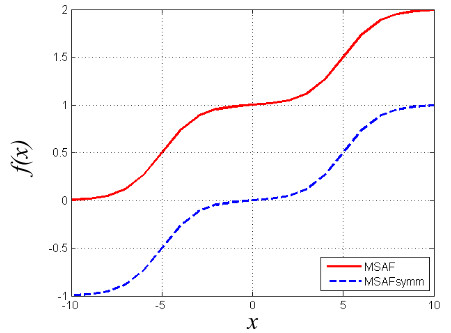MSAF combined with multiple logistic functions and a constant, $r_1$ to have additional output states.

$$f_3 (x) =  \frac{1}{1+exp(-x-r_1)}+ \frac{1}{1+exp(-x)}$$

$$f_4 (x) =  \frac{1}{1+exp(-x-r_1)}+ \frac{1}{1+exp(-x)}$$

### Rectified linear unit (ReLU) and leaky rectified linear unit (LReLU)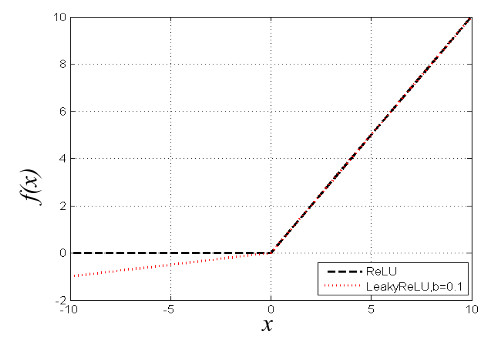ReLU has become a default activation function in DNN because of its simplicity that shortens the training computational time.

Drawback:

• ReLU may not pre-defined boundary. Caused by the neuron that in the negative of the activation function that will no longer be activated throughout the training process.

LReLU can solve this problem.

$$f_5 (x) = \begin{cases} x, & x\gt 0 \ 0, & x\leq 0 \end{cases}$$

$$f_6 (x) = \begin{cases} x, & x\gt 0 \ bx, & x\leq 0 \end{cases}$$

### Exponential linear unit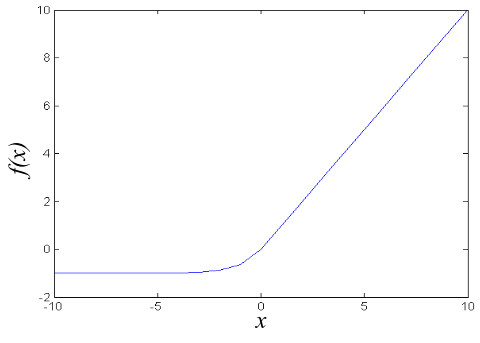ELU reduces bias shift problem, which is defined as the change of a neuron’s mean value due to weights update. ELU has negative values which allow them to push mean unit activations closer to 0 like batch normalization, but with lower computation complexity. Compared to LReLU, ELU has a clear saturation plateau in its negative region.

$$f_7 (\alpha, x) = \begin{cases} \alpha(e^x - 1), & x\lt 0 \ x, & x\ge 0 \end{cases}$$

### SUMMARY OF ACTIVATION FUNCTION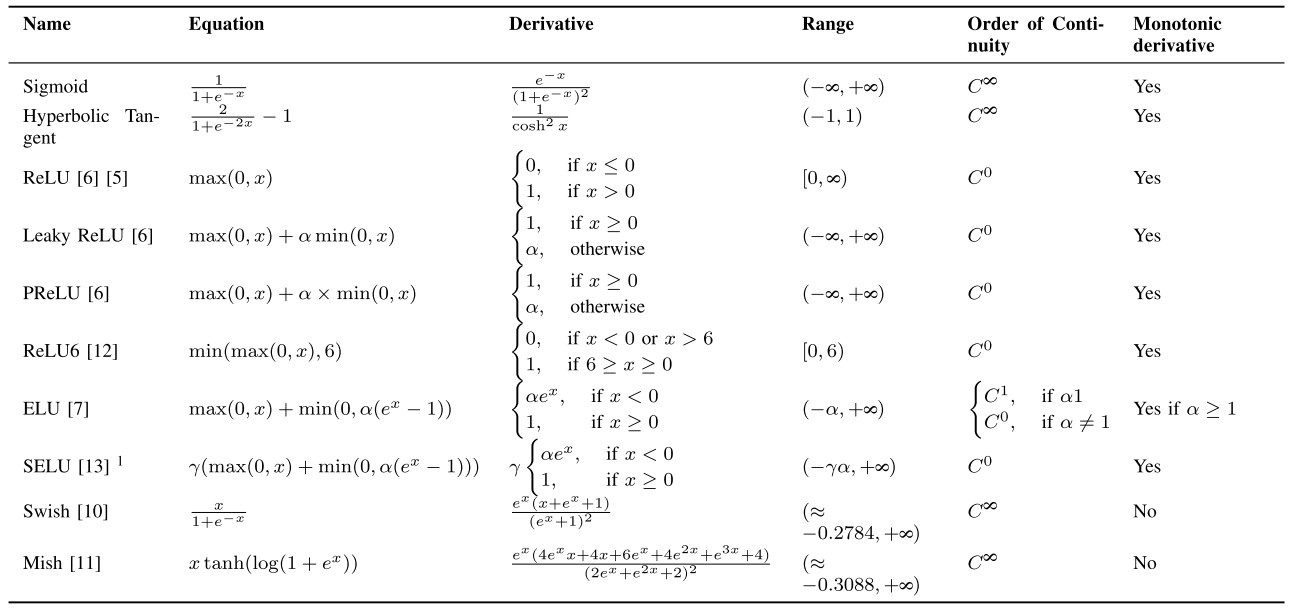## Bias

A bias unit is meant to allow units in your net to learn an appropriate threshold (i.e. after reaching a certain total input, start sending positive activation), since normally a positive total input means a positive activation.

As a switch quantity to control activation function, expressed as $\theta_i$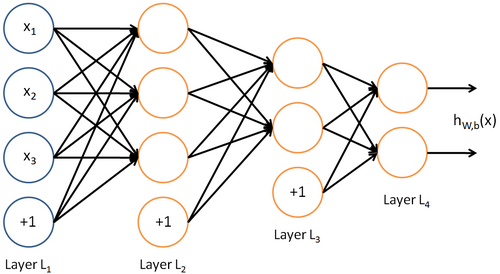# Reference

 Montana, D.J., 1995. Neural network weight selection using genetic algorithms. Intelligent Hybrid Systems, 8(6), pp.12-19.

 Lau, M.M. and Lim, K.H., 2018, December. Review of adaptive activation function in deep neural network. In 2018 IEEE-EMBS Conference on Biomedical Engineering and Sciences (IECBES) (pp. 686-690). IEEE.

 Rasamoelina, A.D., Adjailia, F. and Sinčák, P., 2020, January. A Review of Activation Function for Artificial Neural Network. In 2020 IEEE 18th World Symposium on Applied Machine Intelligence and Informatics (SAMI) (pp. 281-286). IEEE.